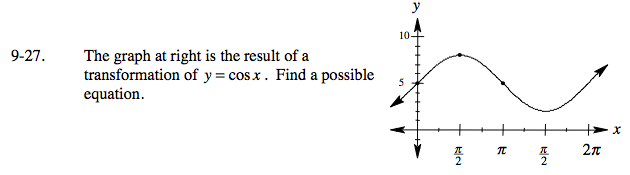### Home > PCT > Chapter Ch9 > Lesson 9.1.2 > Problem9-27

9-27.Focus on the two given points. What can it tell you?

1. The amplitude is 3.
2. The period and frequency is normal.
3. The vertical shift is 5.

$4. \ \ \text{The horizontal shift is } \frac{\pi}{2}.$

(Be careful when you write this in your equation.)

$y = 3\text{cos}\left(x - \frac{\pi}{2}\right) + 5$